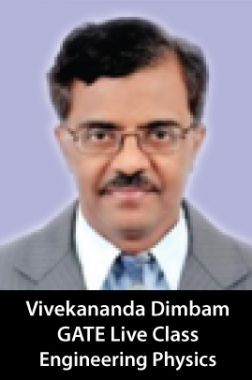•39% Off

# Crash Course Classes Under The Mentorship of Prof. Vivekananda Dimbam, Engineering Physics

By Prof. Vivekananda Dimbam more
111 Views
Offer Price : ₹4,274.05
Course Fees : ₹6,999.00
You will save : ₹2,724.95 after 39% Discount

Upcoming...

Product Specifications

 Publisher Live Tutor All IIT JEE Mains Physics books by Live Tutor Author: Prof. Vivekananda Dimbam Subject Network Analysis, Physics - Signal and system, Power system, Power Electronics No of Classes 39 Available Available in all digital devices

I Am Vivekananda Dimbam, Who is Passionate to TEACH, Design & deploy, review & UPDATE THE Education MODULES of COMPETITIVE SEGMENTS like

IIT GATE / JEE-NEET /CAT / IAS Exams / Science & Maths Olympiad / NTSE & NSTSE Mental ability & Scholasitic Ability Modules

and to successfully administer and manage the activities as Faculty in the Interactive Curriculum of a progressive and vibrant Academic Institutions.

Detail Timing :

Mon - Sat - 09:00 AM - 10:00 AM

We Will Covered All the Section as below :
Section 1: Mathematical Physics  Vector calculus:
linear vector space: basis, orthogonality and completeness; matrices; similarity transformations,  diagonalization,  eigenvalues  and  eigenvectors;  linear  differential  equations:
second order linear differential equations and solutions involving special functions; complex analysis:  Cauchy Riemann  conditions,  Cauchy's  theorem,  singularities,  residue  theorem  and
applications;  Laplace  transform,  Fourier  analysis;  elementary  ideas  about  tensors:  covariant and contravariant tensors
Section 2: Classical Mechanics  Lagrangian formulation: D'Alembert's principle, Euler Lagrange equation, Hamilton's principle, calculus of variations; symmetry and conservation laws; central force motion: Kepler problem
and Rutherford scattering; small oscillations: coupled oscillations and normal modes; rigid body dynamics:  interia  tensor,  orthogonal  transformations,    Euler  angles,  Torque  free  motion  of a symmetric top; Hamiltonian and Hamilton's equations of motion; Liouville's theorem; canonical transformations: action angle variables, Poisson brackets, Hamilton Jacobi equation.
Special  theory  of  relativity: Lorentz  transformations,  relativistic  kinematics,  mass-energy equivalence
Section 3: Electromagnetic Theory  Solutions  of  electrostatic  and  magnetostatic  problems  including  boundary  value  problems; method  of  images;  separation  of  variables;  dielectrics and  conductors;  magnetic  materials;
multipole expansion; Maxwell's equations; scalar and vector potentials; Coulomb and Lorentz gauges; electromagnetic waves in free space, non-conducting and conducting media; reflection
and  transmission  at  normal  and  oblique  incidences;  polarization  of  electromagnetic  waves; Poynting vector, Poynting theorem, energy and momentum of electromagnetic waves; radiation from a moving charge
Section 4: Quantum Mechanics  Postulates of quantum mechanics; uncertainty principle; Schrodinger equation; Dirac BraKet notation,  linear  vectors  and  operators  in  Hilbert  space;  one  dimensional  potentials:  step
potential, finite rectangular well, tunneling from a potential barrier, particle in a box, harmonic oscillator; two and three dimensional systems: concept of degeneracy; hydrogen atom; angular
momentum and spin; addition of angular momenta; variational method and WKB approximation, time  independent  perturbation  theory;  elementary  scattering  theory,  Born  approximation;
symmetries in quantum mechanical systems
Section 5: Thermodynamics and Statistical Physics  Laws  of  thermodynamics;  macrostates  and  microstates;  phase  space;  ensembles;  partition function, free energy, calculation of thermodynamic quantities; classical and quantum statistics;
degenerate  Fermi  gas;  black  body  radiation  and  Planck's  distribution  law;  Bose-Einstein condensation; first and second order phase transitions, phase equilibria, critical point.
Section 6: Atomic and Molecular Physics  Spectra  of  one-and many-electron  atoms;  spin-orbit  interaction:  LS  and  jj  couplings; fine  and hyperfine structures; Zeeman and Stark effects; electric dipole transitions and selection rules;
rotational  and  vibrational  spectra  of  diatomic molecules;  electronic  transitions  in  diatomic molecules,  Franck-Condon  principle;  Raman  effect;  EPR,  NMR,  ESR,  X-ray  spectra; lasers:
Einstein coefficients, population inversion, two and three level systems
Section 7: Solid State Physics  Elements of crystallography; diffraction methods for structure determination; bonding in solids; lattice  vibrations  and thermal  properties  of  solids; free  electron theory;  band  theory  of solids:
nearly   free   electron   and   tight   binding   models;   metals,   semiconductors   and   insulators; conductivity, mobility and effective mass; Optical properties of solids; Kramer's-Kronig relation,
intra-and inter-band transitions; dielectric properties of solid; dielectric function, polarizability, ferroelectricity;  magnetic  properties  of  solids;  dia,  para,  ferro,  antiferro  and  ferri-magnetism,
domains  and  magnetic  anisotropy;  superconductivity:  Type-I  and  Type  II  superconductors, Meissner effect, London equation, BCS Theory, flux quantization
Section 8: Electronics  Semiconductors   in   equilibrium: electron   and   hole   statistics   in   intrinsic   and   extrinsic semiconductors; metal-semiconductor  junctions;  Ohmic  and  rectifying  contacts;  PN  diodes,
bipolar  junction  transistors,  field  effect  transistors;  negative  and  positive  feedback  circuits; oscillators, operational amplifiers, active filters; basics of digital logic circuits, combinational and
sequential circuits, flip-flops, timers, counters, registers, A/D and D/A conversion.
Section 9: Nuclear and Particle Physics  Nuclear radii and charge distributions, nuclear binding energy, electric and magnetic moments; semi-empirical mass formula; nuclear models; liquid drop model, nuclear shell model; nuclear
force and two nucleon problem; alpha decay, beta-decay, electromagnetic transitions in nuclei Rutherford  scattering,  nuclear  reactions,  conservation  laws;  fission  and  fusion;  particle
accelerators and detectors; elementary particles; photons, baryons, mesons and leptons; quark model;  conservation  laws,  isospin  symmetry,  charge  conjugation,  parity  and  time-reversal
invariance

## Engineering Physics Books :

Books & Study Materials